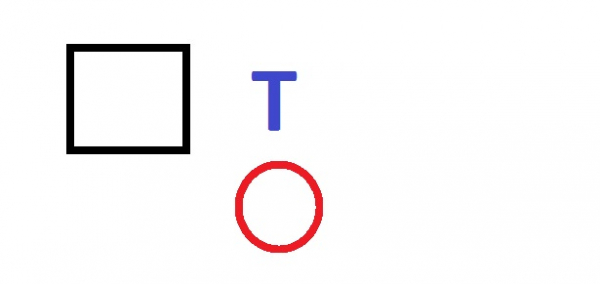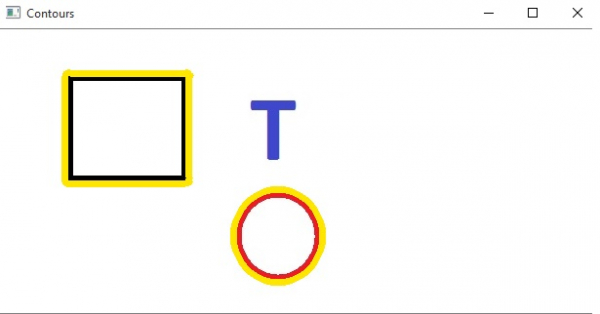# Find and Draw Contours using OpenCV in Python

For the purpose of image analysis we use the Opencv (Open Source Computer Vision Library) python library. The library name that has to be imported after installing opencv is cv2.

In the below example we find the contours present in an image files. Contours help us identify the shapes present in an image. Contours are defined as the line joining all the points along the boundary of an image that are having the same intensity. The findContours function in OPenCV helps us identify the contours. Similarly the drawContours function help us draw the contours. Below is the syntax of both of them.

## Syntax

cv.FindContours(image, mode=CV_RETR_LIST, method=CV_CHAIN_APPROX_SIMPLE)
Where
image is the name of the image
Mode is Contour retrieval mode
Method is Contour approximation method

cv.DrawContours(img, contours, contourIdx, colour, thickness)
Where
image is the name of the image
contours – All the input contours.
contourIdx – Parameter indicating a contour to draw. If it is negative, all the contours are drawn.
color – Color of the contours
thickness is how thick are the lines drawing the contour

## Example

In the below example we use the image below as our input image. Then run the below program to get the contours around it.We can find three shapes in the above diagram. We can draw contours around all or some of them using the below program.

## Example

import cv2
image = cv2.imread(“path to image file”)
# Changing the colour-space
LUV = cv2.cvtColor(image, cv2.COLOR_BGR2LUV)
# Find edges
edges = cv2.Canny(LUV, 10, 100)
# Find Contours
contours, hierarchy = cv2.findContours(edges,cv2.RETR_EXTERNAL, cv2.CHAIN_APPROX_NONE)
# Find Number of contours
print("Number of Contours is: " + str(len(contours)))
# Draw yellow border around two contours
cv2.drawContours(image, contours, 0, (0, 230, 255), 6)
cv2.drawContours(image, contours, 2, (0, 230, 255), 6)
# Show the image with contours
cv2.imshow('Contours', image)
cv2.waitKey(0)

Running the above code gives us the following result −

## Output

Number of Contours found = 3

And we get the below diagram showing the output.